1st grade worksheets are a great way to learn a basic lesson. On 1st grade student learn a simple lesson, such as learning to count and read simple sentences. Besides, students also learn additions and subtraction. Write sentences, and learn to use the proper punctuation also learned in 1st grade. Helping students improve their skill, is teacher task. The teacher can use our free worksheets to improve student skill in reading and writing. Also, we add some first-grade math worksheet to help children learn simple mathematics.

In this article, not all images in HD format. But, some worksheets are in HD in PDF and Doc format, so if you print it you will get a good result.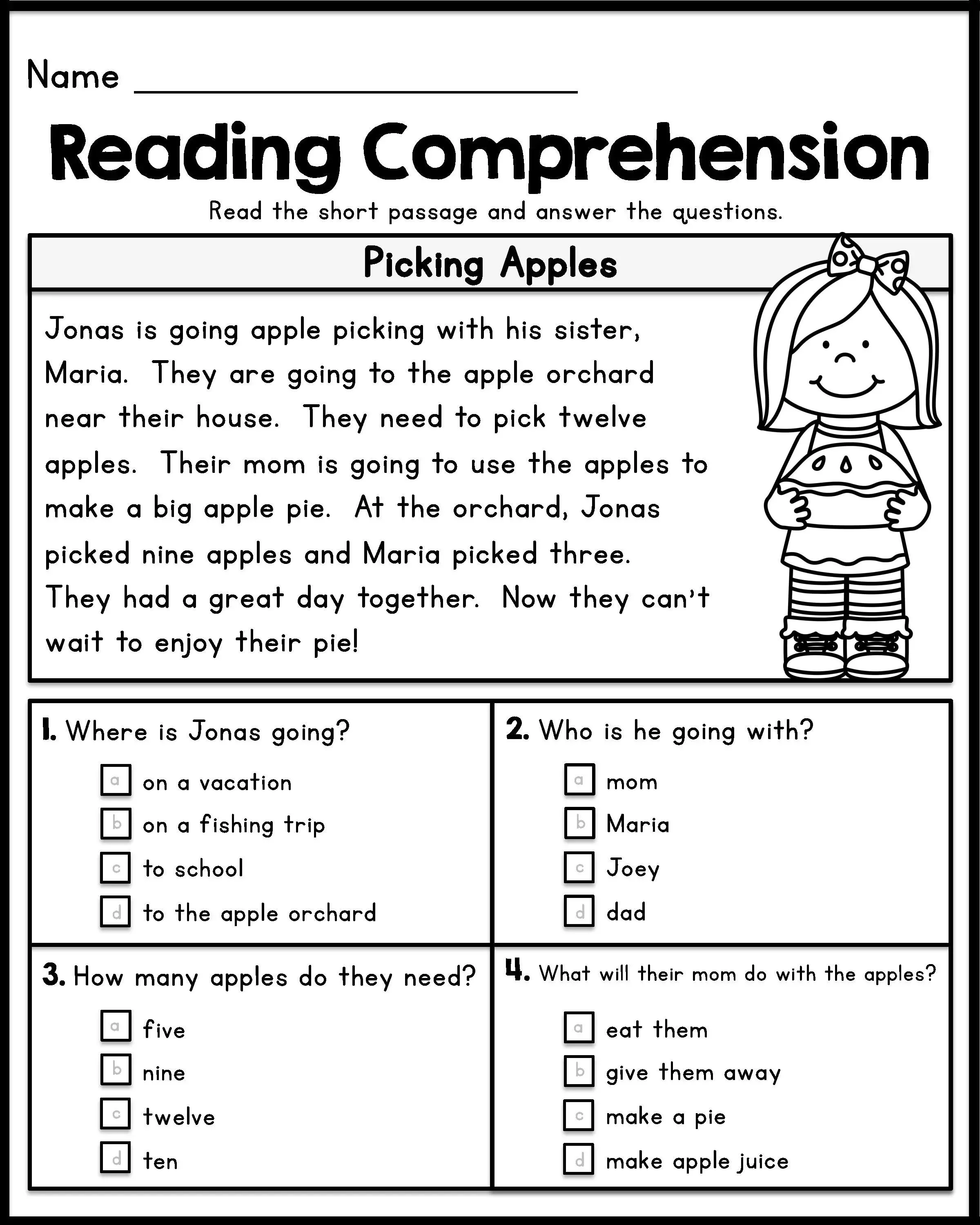Above is simple 1st-grade reading comprehension worksheet with multiple choice question. First, read the short passage, and use it to answer the questions. Second, circle or cross the right answer.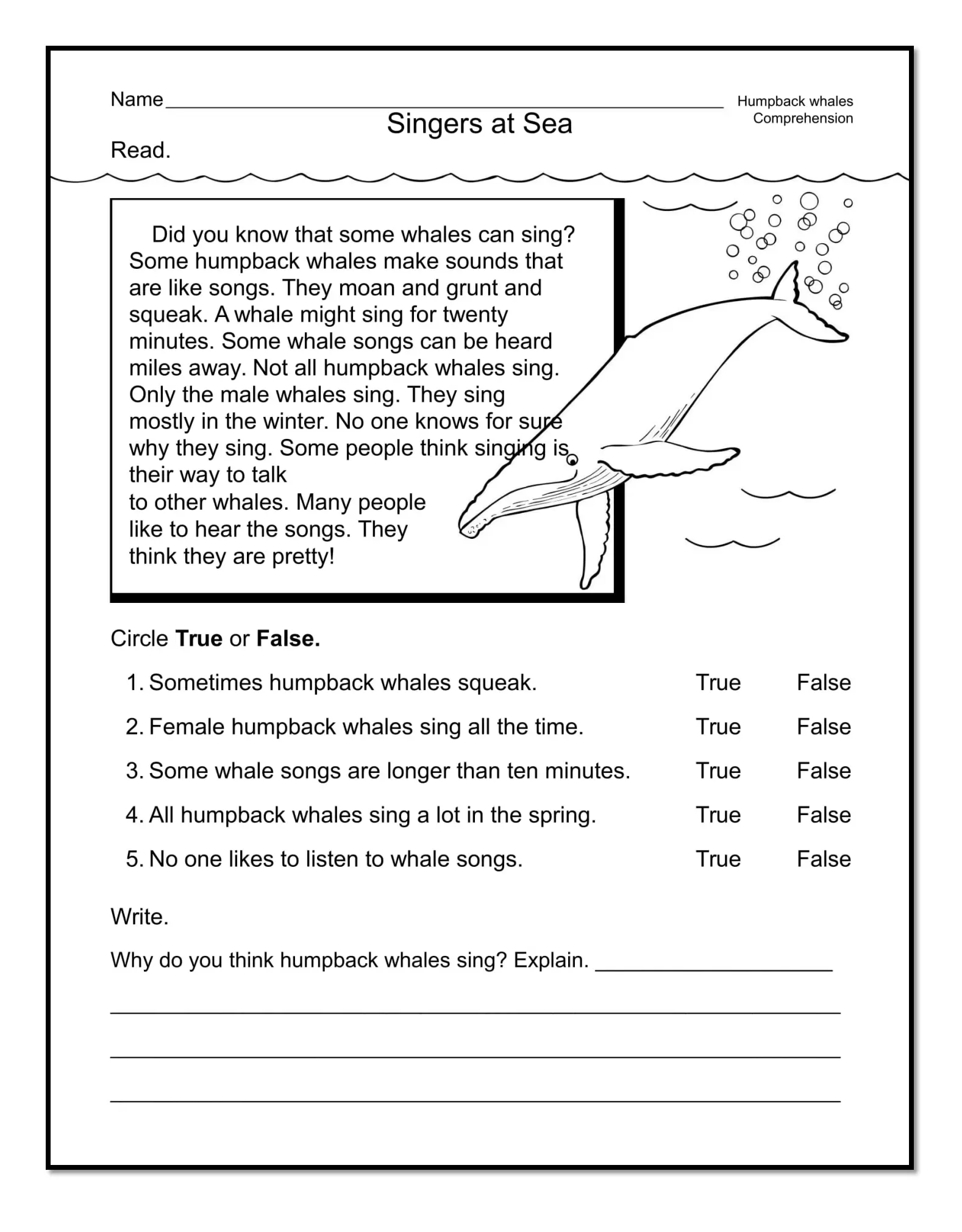In this reading comprehension worksheet, ask your student to read the wholes stories. There are 6 questions, 5 true or false questions, and one essay question to answer.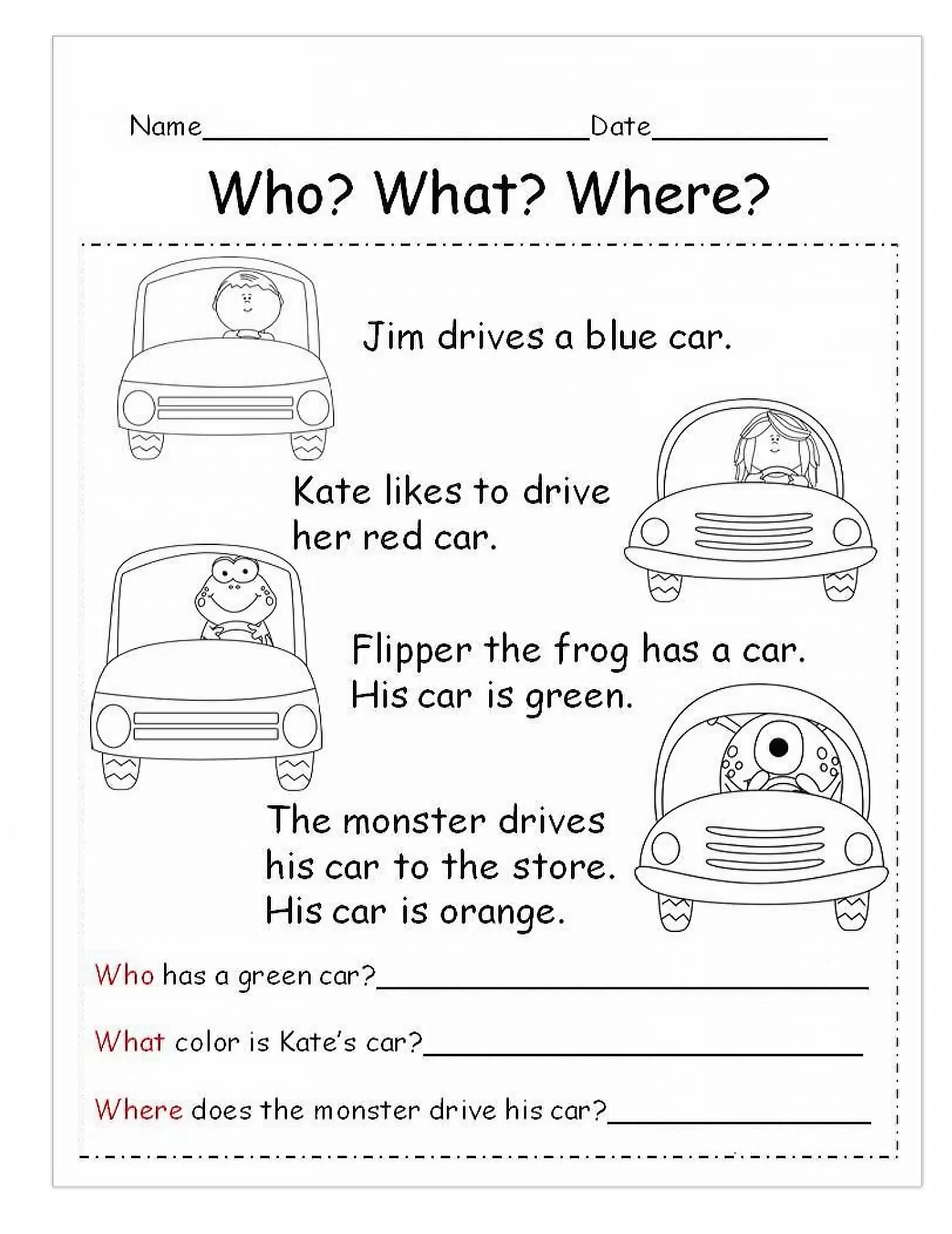Before answering the questions, read the short passage first.

Here students will learn how to write a word or sentence. Writing worksheets including answering a simple question and write a short story.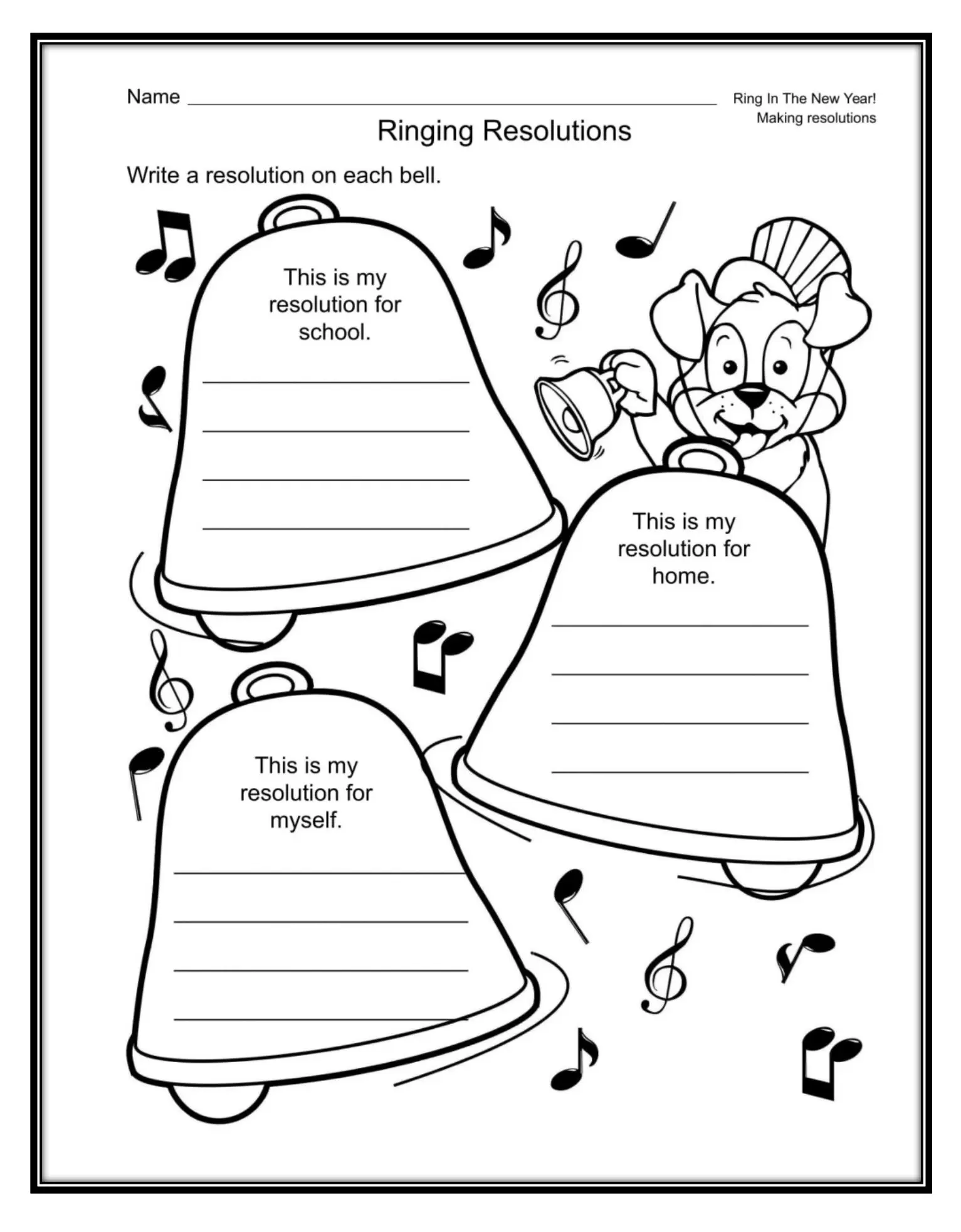To complete writing lessons worksheet above, the student must write 3 new year resolution. one for home, second for school, and third for them self.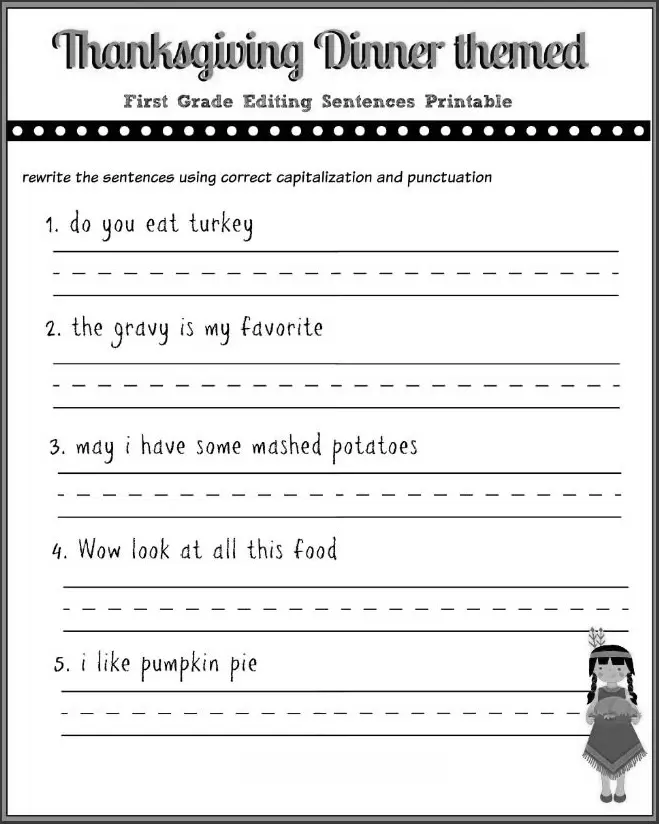Above is a simple writing sentences worksheet with Thanksgiving theme. Use space below each sentence to Rewrite the sentence.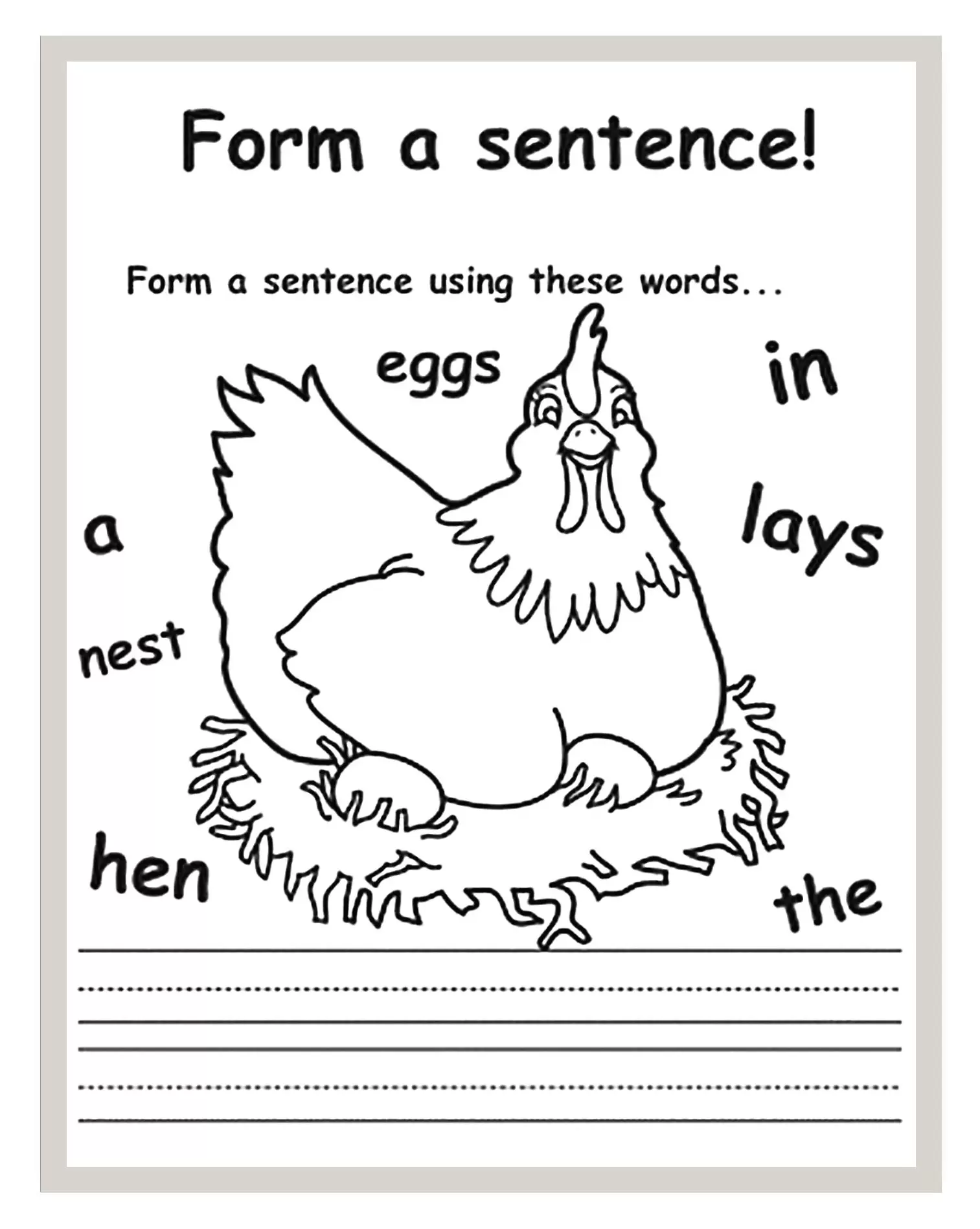There are scramble words on the worksheet above. The student must write a complete sentence from the scramble words. Use the picture on the worksheet, as an illustration, to help form a sentence.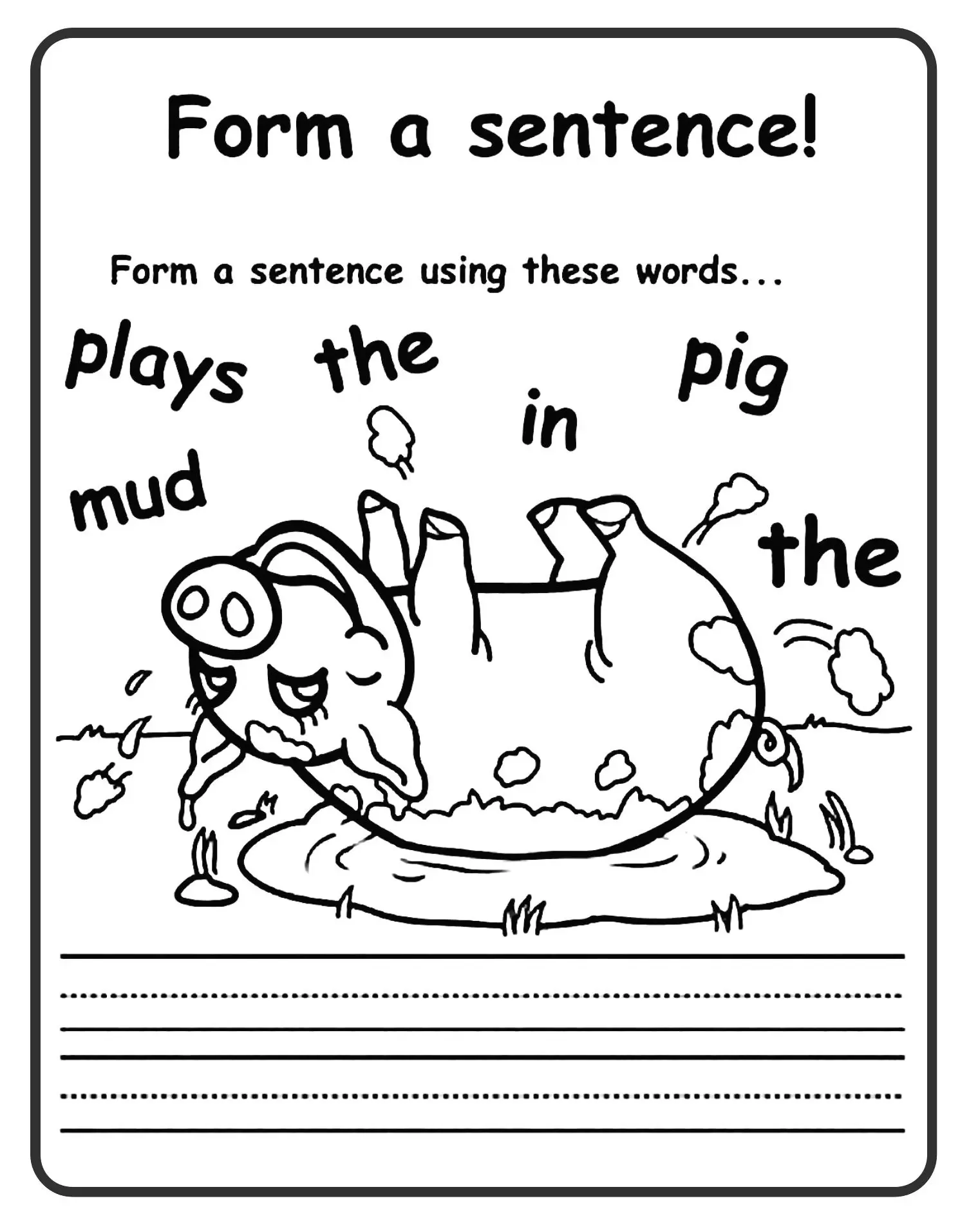Above worksheet is like a previous worksheet, only with pig photo as an illustration. Both worksheets are about build a simple sentence from scrambles words.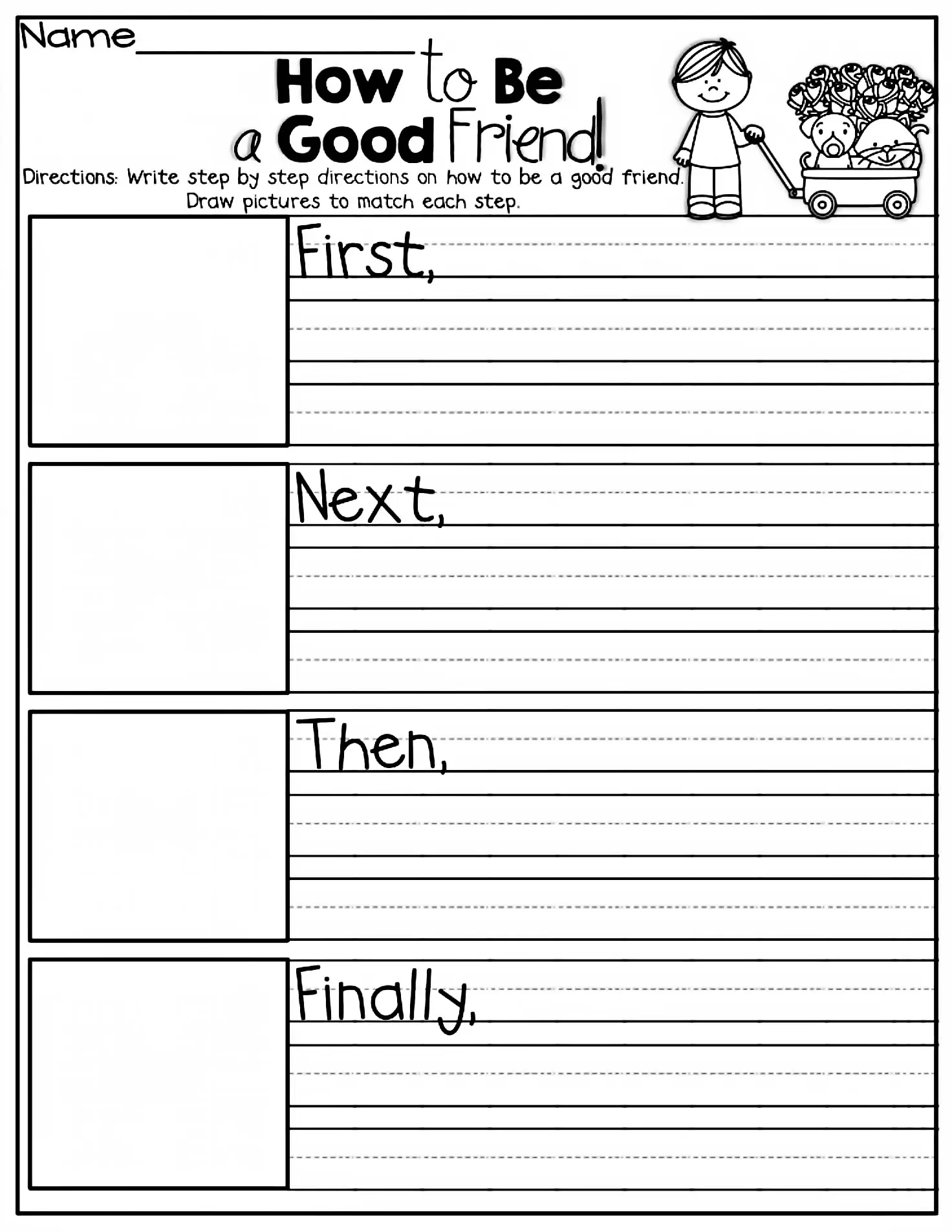As you can see on 1st-grade writing prompts worksheet above, there are two types of answer. First, by drawing pictures on the left box. Second, by making a short sentence. But, the teacher can choose whether students must answer both or choose one.Above is simple 1st grade writing activities worksheet. See link below to download the worksheet.

Math worksheets for 1st grade are include addiction, subtraction, and counting forward worksheets. To illustrate, here we will give some math worksheet for you. Also, we will add more worksheets with an attractive image in the future. Like other worksheets before, we will provide PDF and Microsoft Word format, so you can print it.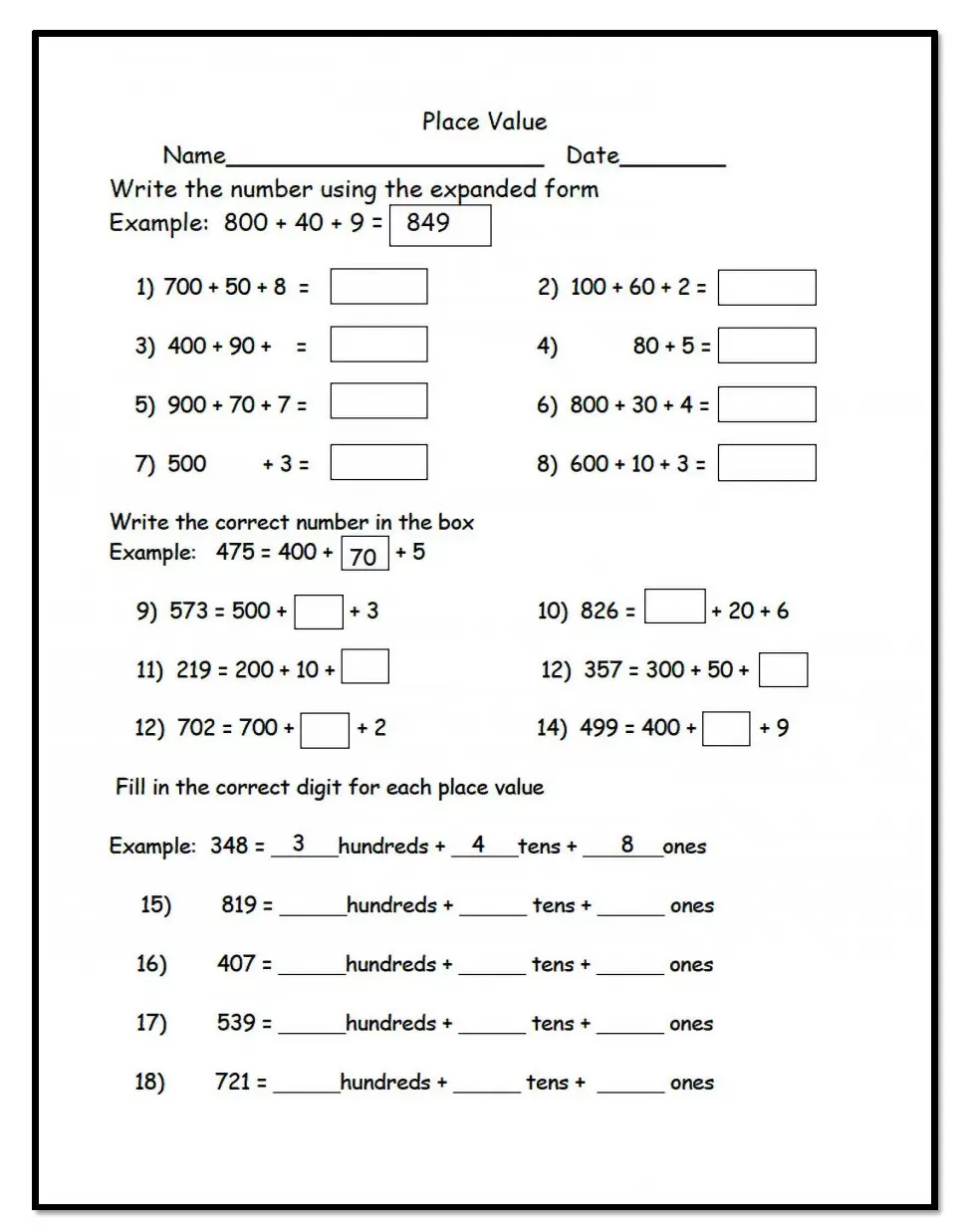The worksheet above is common core worksheet for 1st grade. The student will learn additions and write the correct digit.

Below is a sample of subtraction worksheet. We already include both questions and the answer in the PDF and Word document.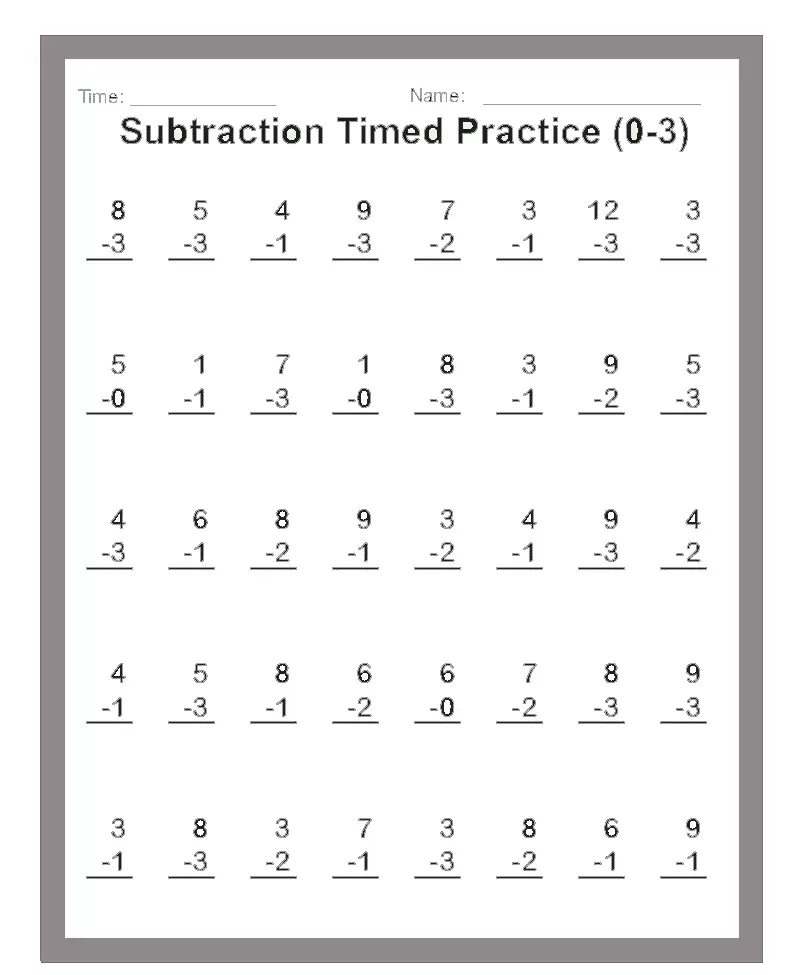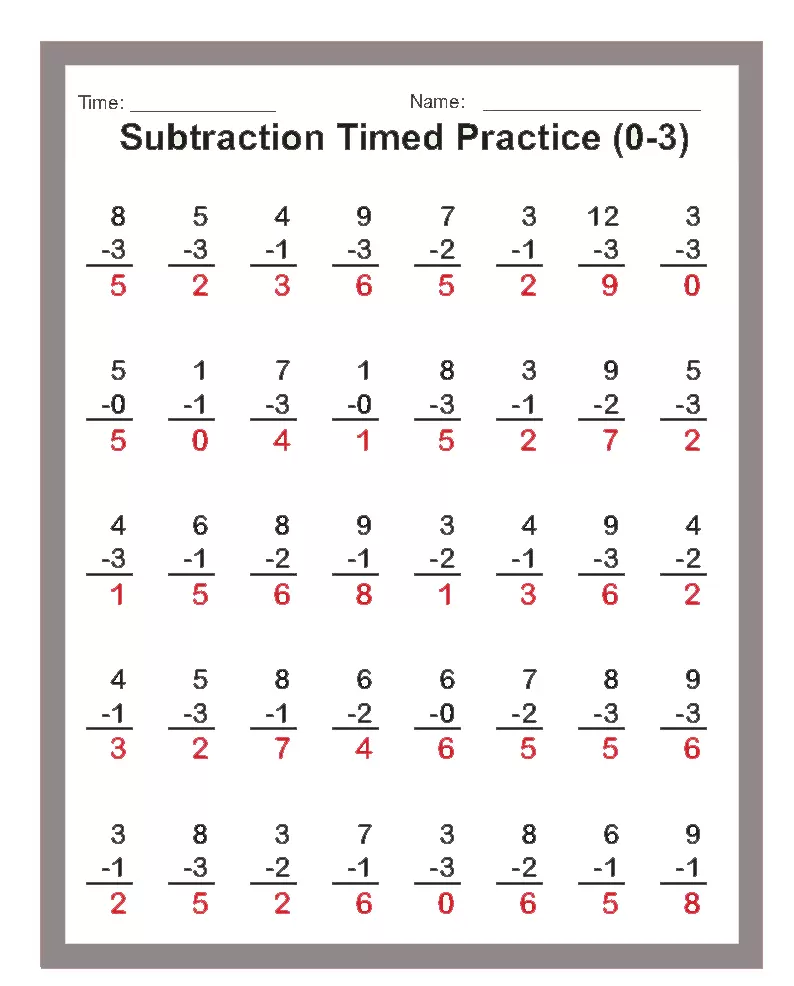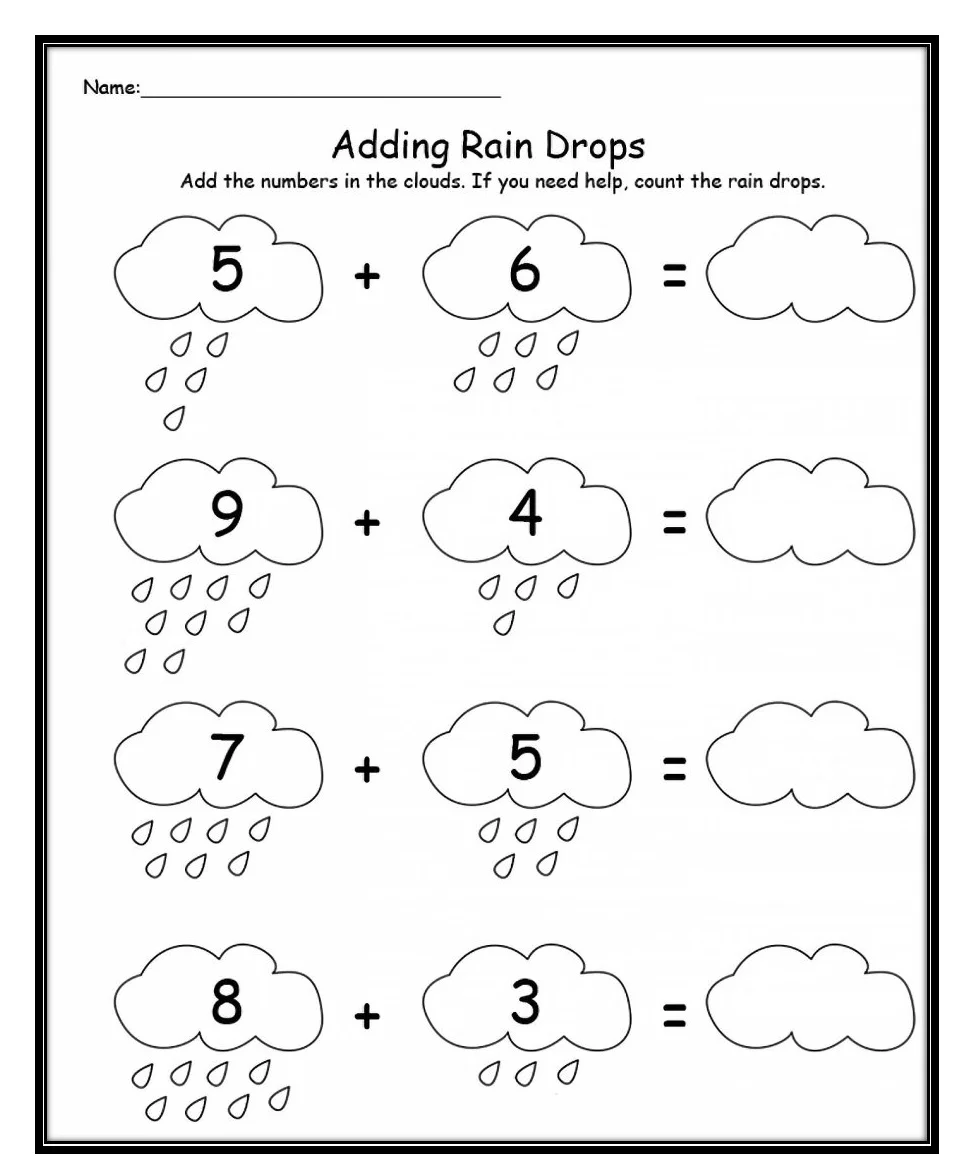Raindrops picture on the worksheet can be use to help the student answer the questions.

1.